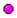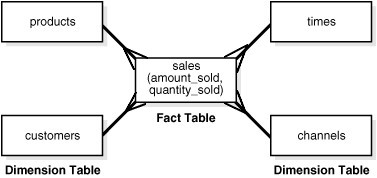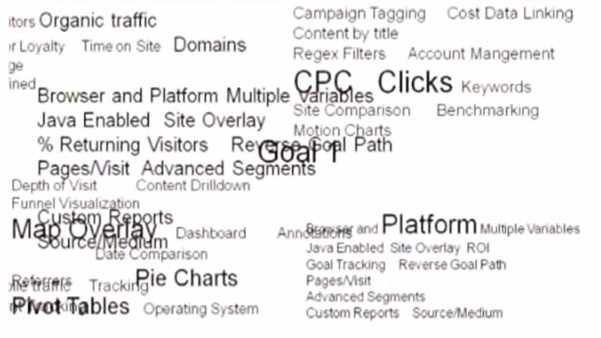# Dimensional Data Modeling - Measure

In a dimensional model, a measure is a quantitative attribute of a fact (in a fact table) that is not a foreign key that creates a relationship to a dimension.

A measure permits to quantify. A calculated and aggregated measure is called a (performance) metrics.

A measure is also known as performance measurement (measurement)

The different types of measurement are categorized into four groups.

As dimensional modeling is the representation of a process, a measure is a quantitative information of an event and is then coupled to the time (dimension) forming a time serie

We cannot improve what we cannot measure, so measure we must.

## Properties

### Data Type

Every measure has a numeric data type.

### Unit

Each measure must have its unit of measurement. If it is a monetary measure, it may have a currency field and if it is a unit measure it may have a field to explain the kind of units used e.g. centimeters, litres, cubic metres etc.

Additivity is crucial because datawarehouse application almost never retrieve a single fact row. The most useful facts are numeric and additive, such as sales amount.

Kimball (1996) defines three levels of additivity.

A Measure fully additive can be aggregated across all dimensions.

Non Additive measures simply can't be added at all. The measures are only valid for a combination of dimension level and are therefore level-based measure.

Example:

• Stock
• and in general all calculations on one specific intersection of the dimension.

A trend for instance is only valid for one month. You can have a positive trend on one month but a negative one on one week.

### Validity

Measures that are valid for:

#### Aggregate

An aggregate measure is the result of a summary on a measure that can be calculated for every level (ie through a classic group by)

The result of an aggregate measure is called a metrics.

#### Level-based

A level-based measure is a column whose values are valid only for a specific combination of level.

A level-based measure may be:

• measured - all non additive measure such as stock, …
• defined - for example, quota, goals are only valid for a combination of level (ie by department and by month)

## Calculated Measure

A calculated measure should be store physically in the database because :

• The cost of a user incorrectly representing gross profit for instance overwhelms the minor incremental storage cost.
• Storing it also ensures that all users and reporting applications refer to profit consistently.

Likewise, some organization want to perform the calculation in the query tool. This works if all users access the data using a common tool (which is seldom the case in our experience).

## Conforming Measure

Conforming Measure means making agreements on common business metrics such as key performance indicators (KPIs) across separated databases so that these numbers can be compared mathematically for calculating differences and ratios.

## Null keys

referential integrity is violated if we put a null in a fact table declared as a foreign key to a dimension table. In addition to the referential integrity alarms, null keys are the source of great confusion to the users because they can't join on null keys.

Avoid null keys in the fact table.

A proper design includes a row in the corresponding dimension table to identify that the dimension is not applicable to the measurement.

## Documentation / Reference

Recommended Pages(Data|State|Operand) Management and Processing

This section is and state management as opposed to code. System that manages data are called database. In a computer, there is two kinds of byte instruction byte and data byte. This section is...BOBJ - Measure =

Measure objects retrieve numeric data that is the result of calculations on data on the database. Measure objects are semantically dynamic: the values they return depend on the objects they are used with....Cube - Olap Database

An OLAP database is an olap application that provide a multidimensional view of the data and are designed to answer analytical questions such as “Why?” and “How?” An OLAP database will contains...Dimensional Data Modeling - A boolean is not a numerical measure but a descriptif attribute

A boolean in dimensional data modeling is not a numerical measure but a discrete attribute A boolean in this article can be represented by: a real boolean (true/false) 0/1 Indicator (Y/N) Really...Dimensional Data Modeling - Degenerate Dimension of Fact dimension (i.e. event/header entity)

In a dimensional model, you may find a dimension table: with a cardinality (distinct value is higher than 10,000). cardinality which has a many-to-one relationship with the fact table close to a one-to-one...Dimensional Data Modeling - Descriptif Attribute (Dimensional Attribute)

A descriptif attribute is class attribute that describe a property or characteristic of a dimension. They are used to label, filter and/or group on. measures Typical attributes for a product dimension...Dimensional Data Modeling - Dimension (Perspective)

A dimension is a part of a dimensional schema and provide the basis for analyzing data through slicing and dicing A dimension is just a set of descriptif attribute that are generally grouped: in a...Dimensional Data Modeling - Dimensional Schemas

This section is dimensional data modeling, That is the building of a cube (hypercube) A dimensional schema is a schema based on dimension. The dimensional schema can modeled: in a relational...Dimensional Data Modeling - Fact Table

A fact table is a table that stores fact where a fact can be seen as the representation of an event. It's also known as: Transactional history Unalterable fact. (The content of this kind of table...Dimensional Data Modeling - Metrics - Key Performance indicators (KPI)

A (performance) metric is an calculated and aggregated measure of activities of: a person, a process, (see ) an event, or an institution organization. Metrics are numeric values. A metric...